NEET  >  Test: Electrostatic Potential due to a Point Charge

# Test: Electrostatic Potential due to a Point Charge

Test Description

## 10 Questions MCQ Test Physics Class 12 | Test: Electrostatic Potential due to a Point Charge

Test: Electrostatic Potential due to a Point Charge for NEET 2023 is part of Physics Class 12 preparation. The Test: Electrostatic Potential due to a Point Charge questions and answers have been prepared according to the NEET exam syllabus.The Test: Electrostatic Potential due to a Point Charge MCQs are made for NEET 2023 Exam. Find important definitions, questions, notes, meanings, examples, exercises, MCQs and online tests for Test: Electrostatic Potential due to a Point Charge below.
Solutions of Test: Electrostatic Potential due to a Point Charge questions in English are available as part of our Physics Class 12 for NEET & Test: Electrostatic Potential due to a Point Charge solutions in Hindi for Physics Class 12 course. Download more important topics, notes, lectures and mock test series for NEET Exam by signing up for free. Attempt Test: Electrostatic Potential due to a Point Charge | 10 questions in 10 minutes | Mock test for NEET preparation | Free important questions MCQ to study Physics Class 12 for NEET Exam | Download free PDF with solutions
 1 Crore+ students have signed up on EduRev. Have you?
Test: Electrostatic Potential due to a Point Charge - Question 1

### Electric potential due to a point charge q at a distance r from the point is _______ (in the air).

Detailed Solution for Test: Electrostatic Potential due to a Point Charge - Question 1

Force on a unit point charge kept at a distance r from the charge = q/r2

Therefore, work done to bring that point charge through a small distance dr = q/r2 * (-dr). Therefore, the potential of that point is =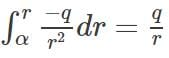.

Test: Electrostatic Potential due to a Point Charge - Question 2

### Calculate electric potential due to a point charge of 10C at a distance of 8cm away from the charge.

Detailed Solution for Test: Electrostatic Potential due to a Point Charge - Question 2

In the SI system, electric potential due to a point charge at a distance r is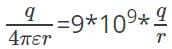. Substituting the values, we get potential =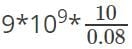V = 1.125*1012V. Though in practice, this huge value of electric potential is not present.

Test: Electrostatic Potential due to a Point Charge - Question 3

### What is the amount of work done to bring a charge of 4*10-3C charge from infinity to a point whose electric potential is 2*102V?

Detailed Solution for Test: Electrostatic Potential due to a Point Charge - Question 3

Work done = potential*charge by definition. We know that the potential of a point is the amount of work done to bring a unit charge from infinity to a certain point. Therefore, work done W=q*V=4*10-3*200J=0.8J. The work done is positive in this case.

Test: Electrostatic Potential due to a Point Charge - Question 4

Two point charges 10C and -10C are placed at a certain distance. What is the electric potential of their midpoint?

Detailed Solution for Test: Electrostatic Potential due to a Point Charge - Question 4

Electric potential is a scalar quantity and its value is solely dependent on the charge near it and the distance from that charge. In this case, the point is equidistant from the two point charges and the point charges have the same value but opposite nature. Therefore equal but opposite potentials are generated due to the charges and hence the net potential at midpoint becomes zero.

Test: Electrostatic Potential due to a Point Charge - Question 5

What is the electric potential of the system?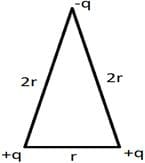Detailed Solution for Test: Electrostatic Potential due to a Point Charge - Question 5

Electric potential between +q and +q is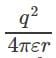and electric potential between two charges +q and –q is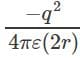. Therefore the electric potential of the system is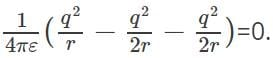Electric potential is a scalar quantity and thus the system becomes zero-potential-system.

Test: Electrostatic Potential due to a Point Charge - Question 6

A small charge q is rotated in a complete circular path of radius r surrounding another charge Q. The work done in this process is _________

Detailed Solution for Test: Electrostatic Potential due to a Point Charge - Question 6

We know that electric potential does not depend on the path, i.e. it is a state function. Therefore if we rotate a charge around another in a complete circular path, the potential energy of its initial and final points is the same. Therefore, there is no change of potential energy of the charge and hence net work done on the charge is zero.

Test: Electrostatic Potential due to a Point Charge - Question 7

Two plates are kept at a distance of 0.1m and their potential difference is 20V. An electron is kept at rest on the surface of the plate with lower potential. What will be the velocity of the electron when it strikes another plate?

Detailed Solution for Test: Electrostatic Potential due to a Point Charge - Question 7

Increase in potential energy of the electron when it strikes another plate = Potential difference*charge of electron=20*1.6*10-19 J=3.201*10-18J and as the electron was at rest initially, this energy will be converted to kinetic energy completely. Therefore, 0.5*mass of electron*(velocity)2=3.201*10-18J. Substituting m=9.11*10-31kg, we get v=2.65*106 m/s.

Test: Electrostatic Potential due to a Point Charge - Question 8

Electric field intensity and electric potential at a certain distance from a point charge is 32 N/C and 16 J/C. What is the distance from the charge?

Detailed Solution for Test: Electrostatic Potential due to a Point Charge - Question 8

Electric field due to a charge q at a distance r is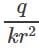and electric potential at that point is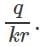Therefore,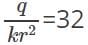and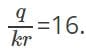Dividing these two equations, we get r=0.5m. If the medium is air, k=1. Thus we can get the value of q=0.89 C.

Test: Electrostatic Potential due to a Point Charge - Question 9

Three charges –q, Q and –q are placed in a straight line maintaining equal distance from each other. What should be the ratio q/Q so that the net electric potential of the system is zero?

Detailed Solution for Test: Electrostatic Potential due to a Point Charge - Question 9

Let the distance between any two charges is d. Therefore the net potential energy of the system is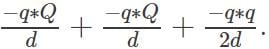But the total energy of the system is zero. So, -qQ-qQ+ q2/2

=0 that means q=4Q, i.e. q/Q=4.

Test: Electrostatic Potential due to a Point Charge - Question 10

Three positive charges are kept at the vertices of an equilateral triangle. We can make the potential energy of the system zero by adjusting the amount of charges. This statement is ______

Detailed Solution for Test: Electrostatic Potential due to a Point Charge - Question 10

Electric potential is a positive quantity if both the charges are positive. In this case, all the three charges are positive; hence there is no negative term in the total energy term. Therefore, we cannot make the total energy of the system zero by adjusting the values of charge. But it would be possible if one of the charges were positive.

## Physics Class 12

157 videos|452 docs|213 tests
 Use Code STAYHOME200 and get INR 200 additional OFF Use Coupon Code
Information about Test: Electrostatic Potential due to a Point Charge Page
In this test you can find the Exam questions for Test: Electrostatic Potential due to a Point Charge solved & explained in the simplest way possible. Besides giving Questions and answers for Test: Electrostatic Potential due to a Point Charge, EduRev gives you an ample number of Online tests for practice

## Physics Class 12

157 videos|452 docs|213 tests

### How to Prepare for NEET

Read our guide to prepare for NEET which is created by Toppers & the best Teachers